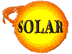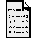How is hydrogen fused into helium?(by Amara Graps)

In the Sun the primary process is called the "proton-proton" chain. In this reaction four protons (these are the hydrogen nuclei) are fused into one helium nucleus (also called an "alpha particle"). An alpha particle has about 1% less mass than four protons. The rest of the mass is turned into gamma rays - high energy photons. The Sun converts about 5 million tons of mass into energy every second. (Don't worry, there's enough for a long time!)

Here is the reaction in chemical notation

4 1H + 2 e --> 4He + 2 neutrinos + 6 photons
Fusion can only happen when they can get close to each other. It is a process that actually has several stages because the protons ("1H") both have a positive charge and at lower temperatures they repel each other. But because the gas in the center of the Sun is so hot, the protons can hit each other with high speed. When they get close to each other a natural force in nature for tiny distances, the strong nuclear force, can bind the particles together. Several other intermediate steps take place where electrons and antielectrons annihilate each other, more protons collide into each other and a helium atom is made and with the helium and neutrons and protons bouncing around in the hot gas, when they get close to each other, new particles are created.

So you see our one equation above has many steps! In chemical notation it looks like:

1H + 1H --> 2H + antielectron + neutrino
1H + 1H --> 2H + antielectron + neutrino
electron + antielectron --> photon + photon
electron + antielectron --> photon + photon
2H + 1H --> 3He + photon
2H + 1H --> 3He + photon
3He + 3He --> 4He + 1H+ 1H
Or Rewriting:
6 1H + 2 e --> 4He + 2 1H + 2 neutrinos + 6 photons
Which is just our original equation above:
4 1H + 2 e --> 4He + 2 neutrinos + 6 photonsBack to the SOLAR CenterComments?